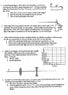# 5 Questions dealing with Optics, image included

• Pharrett

#### Pharrett

1. A real inverted image I of an object O is formed by a certain lens (not shown); the object—image separation
is d = 40.0 cm, measured along the central axis of the lens. The image is just half the size of the object,
a. What kind of lens must be used to produce this image?
b. How far from the object must the lens be placed?
c. What is the focal length of the lens?

Relevant equations:

1/f=1/q+1/p

Attempt at solution:
Found p=40-q
1/q + 1/40-q = 1/f
Used the Sign of f to decide the lens was convex
Used final q to determine the final p and used Pf as distance between object and lens

2.
White light, traveling parallel to the base of a prism with an apex angle of Ho, and enters the prism near the top Apex angle. After the light exits the prism on opposite face, what is the angle (angular spread) between the red light and light if the index of refraction is 1.51 for red light and 1.53 for violet light.

Relevant Equations:

θ₁=θ₂
Sinθ₁=Sinθ₂

Attempt at solution:
Found θ₁ to be 15°(using symmetry of the prism 90-75°), used that to find θ₂ to be 9.xxx°
Then used the parallel light and normal rays and 75°(see image) to find an angle of 15° and 105° for the triangle and then found a third angle of 60° which I used to get the θ₃=30°.
Next I used 1.51 for red to find θf=49.xxxxx°

I did the same thing using 1.53 for purple and found θf=49.xxxxx°
Then, I subtracted the PURPLE from the RED and got 0.88xxxxx° has my angular spread.

3.
If the distance between the first and tenth minima of a double-slit pattern is 18.0mm and the slits are separated by 0.150 mm with the screen 50.0 cm from the slits,
a. what is the wavelength of the light used? (assume experiment is in air)
b. What would be the spacing between the first and tenth minima if this same experiment (with same light source) was done underwater (n = 1.33)?

Relevant Equations:

Δy=(Δm+½)λL/dn

Attempt at Solution:
Using the above equation and Δm=9 and n=1, i found λ
then i used λ and n=1.33 to find Δy for part b).

4.
Light of wavelength 440 nm passes through a double slit, yielding a diffraction pattern whose graph of intensity versus angular position 0 is shown in the figure.[B/][B\]
Calculate
a. the slit width.
b. the slit separation.

Relevant Equations:

I/Io=[Sin(β/2)/(β/2)]² x Cos²(φ/2)
dSinθ=mλ;bright double slit
m=4(see image for graph)
aSinθ=mλ; dark single slit
d/a=m

Attempt at Solution:

I found m to be 4 by using the graph and counting the intensities starting with m=0 at the center.
I also used the graph to see that there appears to be a missing maximum and Sinθ=5
I used aSinθ=mλ with m =4 to find a=20.xxxnm
then I used d/a=m with m=4 to find d=80.xxxxnm

5.
An object is 20 cut from a convex lens. A concave lens is 15 cm from the convex lens. For both lenses the magnitude of their focal lengths is 7.5cm.
a. Row far is the object from the final image?
b. With the spacing describe above, if the concave lens is moving with an instantaneous speed of 2m/s toward the convex lens, what is the speed and direction of the final image?

Relevant Equations:

1/f=1/p+1/q
M=-q/p=h'/h
d/dt(1/f=1/p+1/q)→0=-1/p² (dP/dt) + -1/q² (dQ/dt)
dP/dt=200cm/s

Attempt at Solution:

I found the first image using convex lens sign conventions
then I found the final image using Concave lens sign conventions
my final answer was -2.32xxxxx cm for of the final image,
my answer to a was 32.32 cm;
Then for b) using only my final equation for the secon image, I took the derivative and got the above equation, Solved for (dQ/dt) and found it to be -102.xxxx cm/s traveling toward the convex lens.

#### Attachments

• Physics 123 Exam 3 Optics 2016.pdf
278.5 KB · Views: 343
•Physics 123 Exam 3 Optics.jpg
45.3 KB · Views: 736
Last edited by a moderator: